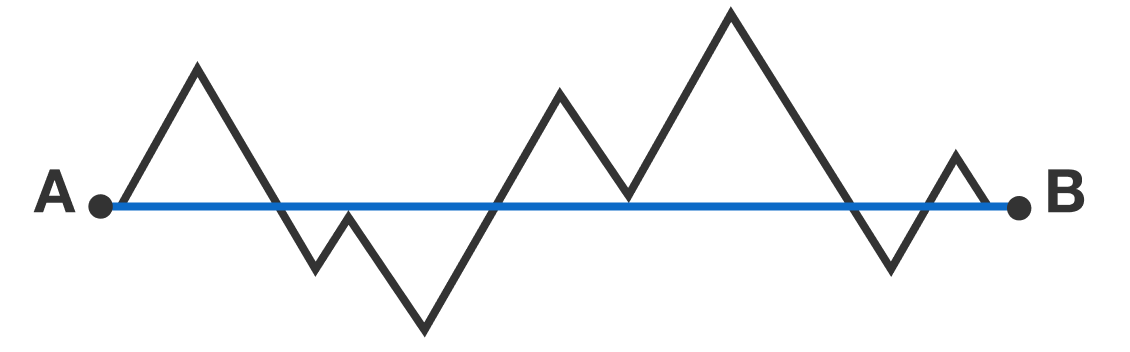# Cardiac Monitoring

Geometry Level 1The polygonal path connecting points A and B forms 7 equilateral triangles.

If the distance between point A to point B is 20 units, then how long is the distance of the path (in units)?

×